# JavaScript: For loop that will iterate from 0 to 15 to find even and odd numbers

## JavaScript Conditional Statement and loops: Exercise-5 with Solution

Write a JavaScript for loop that will iterate from 0 to 15. For each iteration, it will check if the current number is odd or even, and display a message to the screen.

Sample Output:
"0 is even"
"1 is odd"
"2 is even"
----------
----------

Calculate a Even Number: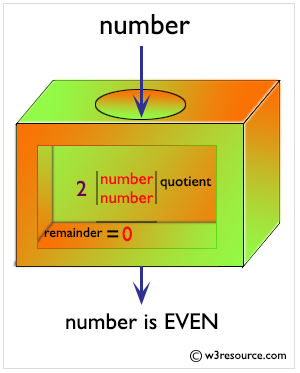Even Numbers between 1 to 100: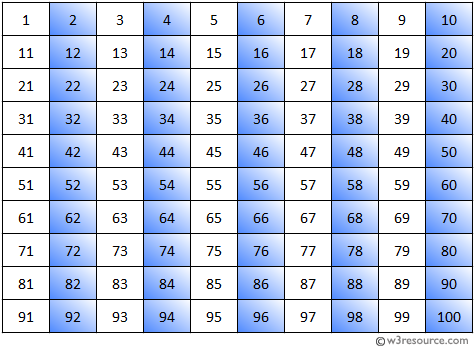Calculate a Odd Number: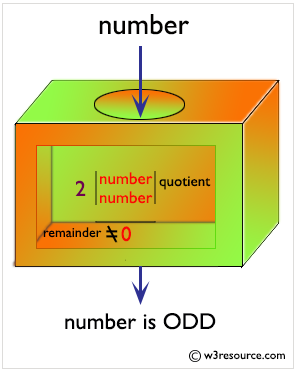Odd Numbers between 1 to 100: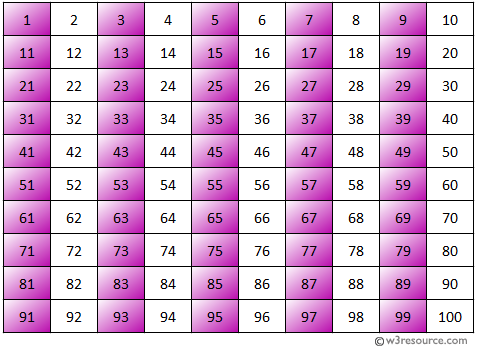Sample Solution:-

HTML Code:

``````
<!DOCTYPE html>
<html>
<meta charset=utf-8 />
<title>For loop that will iterate from 0 to 15</title>
<body>

</body>
</html>
```
```

JavaScript Code:

``````
for (var x=0; x<=15; x++) {
if (x === 0) {
console.log(x +  " is even");
}
else if (x % 2 === 0) {
console.log(x + " is even");
}
else {
console.log(x + " is odd");
}
}
```
```

Sample Output:

```0 is even
1 is odd
2 is even
3 is odd
4 is even
5 is odd
6 is even
7 is odd
8 is even
9 is odd
10 is even
11 is odd
12 is even
13 is odd
14 is even
15 is odd
```

Flowchart: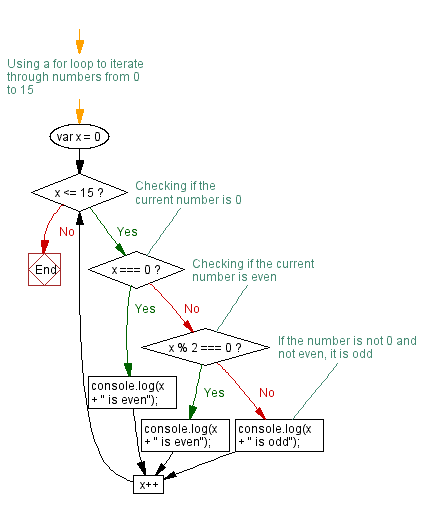Live Demo:

See the Pen javascript-conditional-statements-and-loops-exercise-5 by w3resource (@w3resource) on CodePen.

Improve this sample solution and post your code through Disqus

What is the difficulty level of this exercise?

﻿

New Content: Composer: Dependency manager for PHP, R Programming